#1年前
458409

## 前言

• Java内存模型，可见性问题
• CAS
• HashMap底层原理

1. 更灵活的运用ConcurrentHashMap
2. 欣赏并发编程大师Doug Lea的作品，源码中有很多值得我们学习的并发思想，要意识到，线程安全不仅仅只是加锁

• ConcurrentHashMap是怎么做到线程安全的？
• get方法如何线程安全地获取key、value？
• put方法如何线程安全地设置key、value？
• size方法如果线程安全地获取容器容量？
• 底层数据结构扩容时如果保证线程安全？
• 初始化数据结构时如果保证线程安全？
• ConcurrentHashMap并发效率是如何提高的？
• 和加锁相比较，为什么它比HashTable效率高？

## 相关概念

### Amdahl定律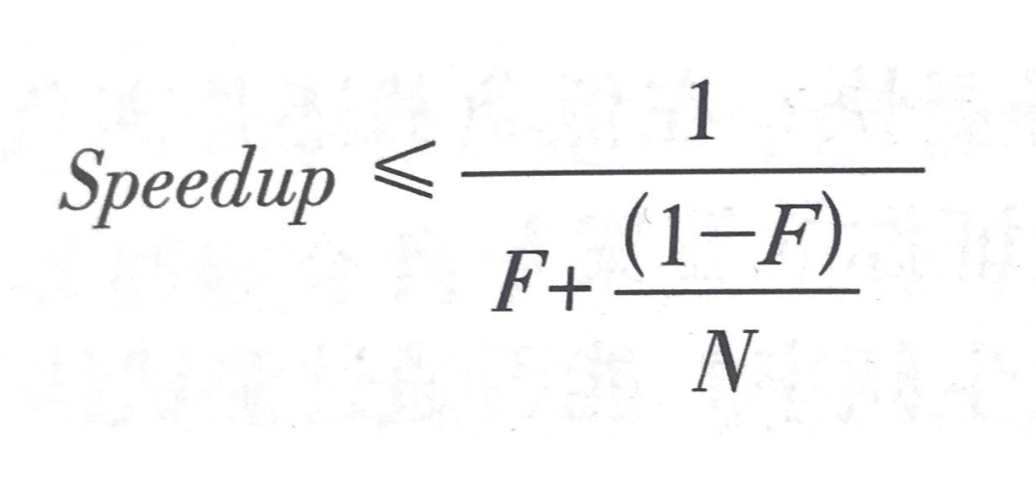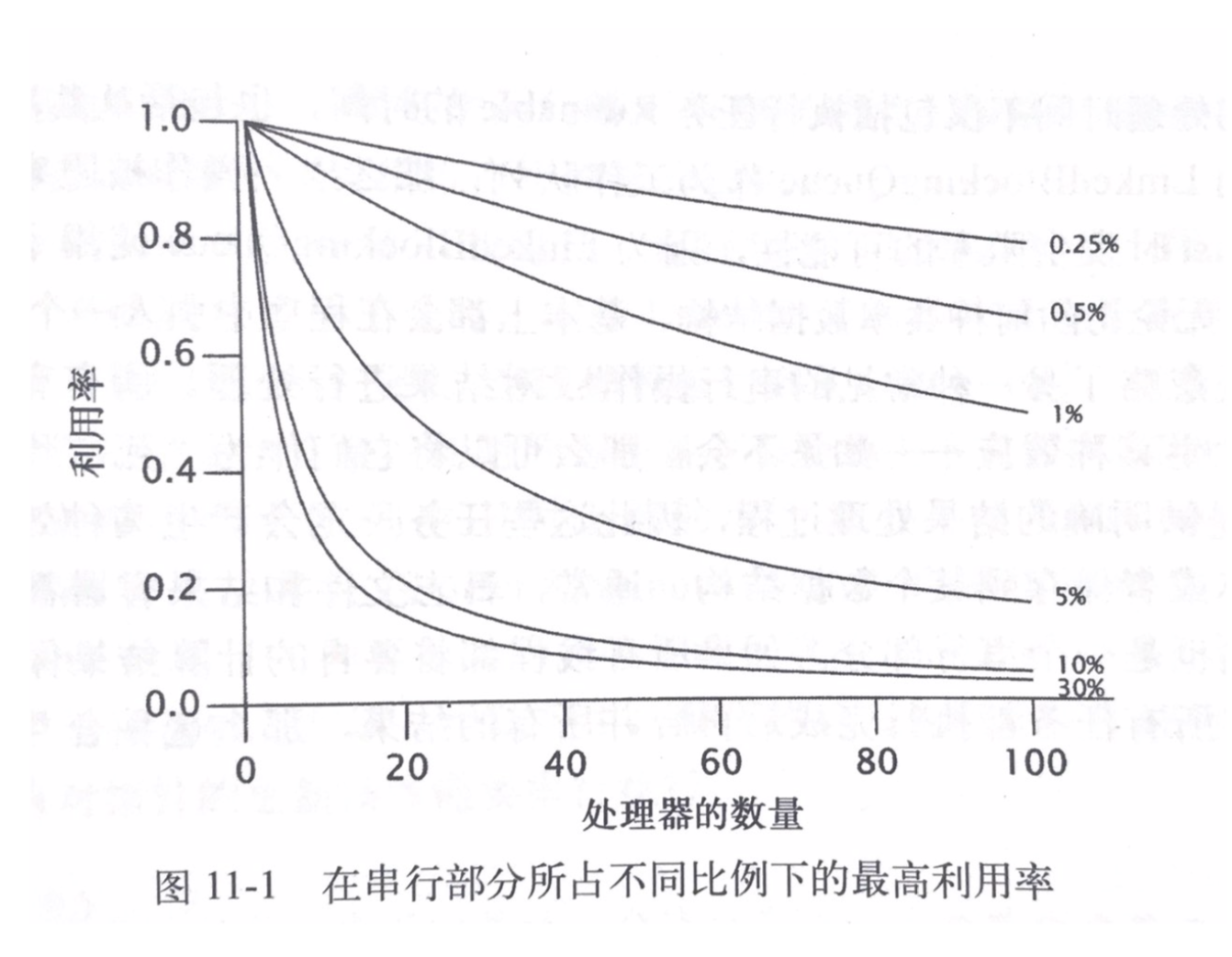## 初始化数据结构时的线程安全

HashMap的底层数据结构这里简单带过一下，不做过多赘述：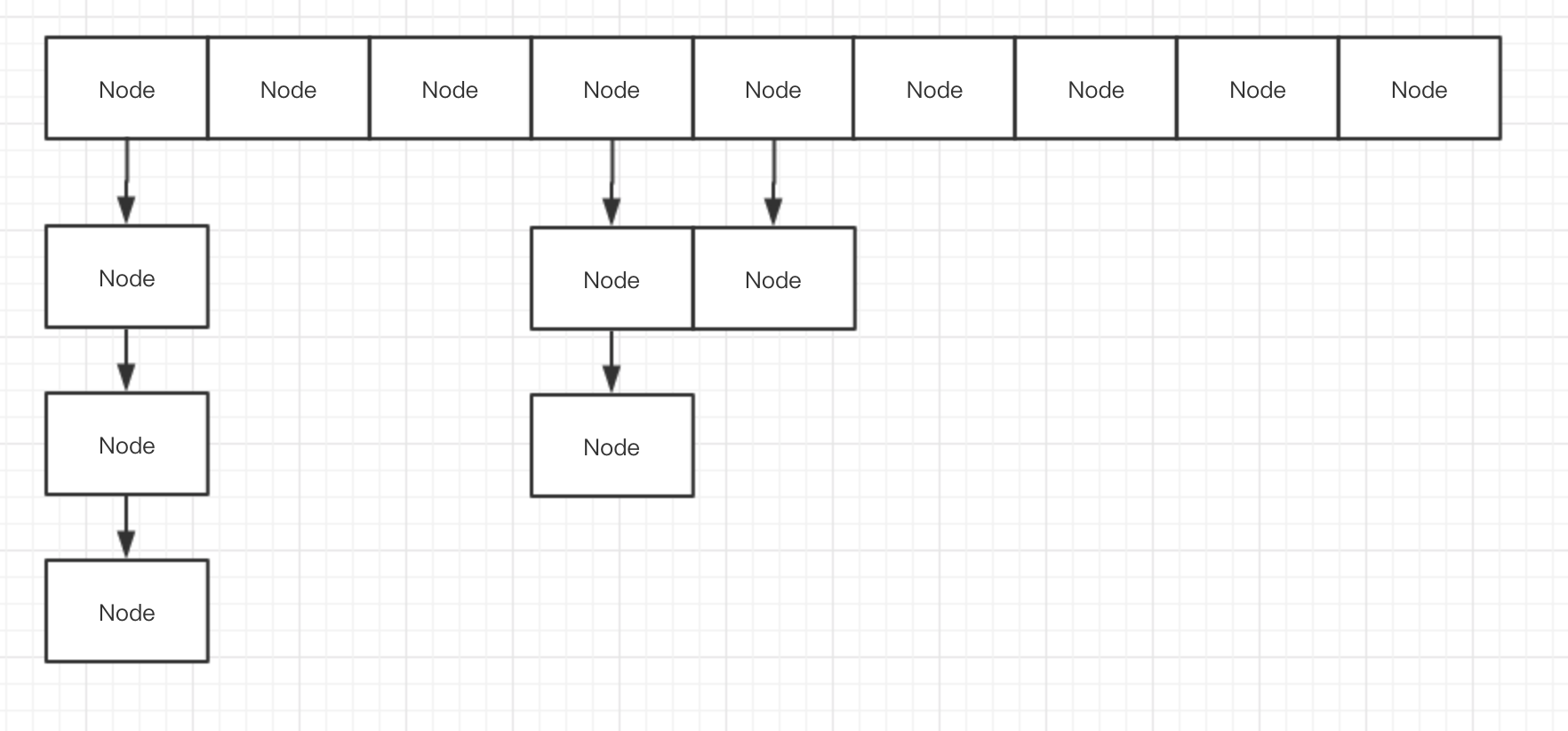``````static class Node<K,V> implements Map.Entry<K,V> {

final int hash;
final K key;
volatile V val;
volatile Node<K,V> next;
...
}
``````

``````final V putVal(K key, V value, boolean onlyIfAbsent) {

if (key == null || value == null) throw new NullPointerException();
int binCount = 0;
for (Node<K,V>[] tab = table;;) {

Node<K,V> f; int n, i, fh;
//判断Node数组为空
if (tab == null || (n = tab.length) == 0)
//初始化Node数组
tab = initTable();
...
}
``````

``````private final Node<K,V>[] initTable() {

Node<K,V>[] tab; int sc;
//每次循环都获取最新的Node数组引用
while ((tab = table) == null || tab.length == 0) {

//sizeCtl是一个标记位，若为-1也就是小于0，代表有线程在进行初始化工作了
if ((sc = sizeCtl) < 0)
//让出CPU时间片
Thread.yield(); // lost initialization race; just spin
//CAS操作，将本实例的sizeCtl变量设置为-1
else if (U.compareAndSwapInt(this, SIZECTL, sc, -1)) {

//如果CAS操作成功了，代表本线程将负责初始化工作
try {

//再检查一遍数组是否为空
if ((tab = table) == null || tab.length == 0) {

//在初始化Map时，sizeCtl代表数组大小，默认16
//所以此时n默认为16
int n = (sc > 0) ? sc : DEFAULT_CAPACITY;
@SuppressWarnings("unchecked")
//Node数组
Node<K,V>[] nt = (Node<K,V>[])new Node<?,?>[n];
//将其赋值给table变量
table = tab = nt;
//通过位运算，n减去n二进制右移2位，相当于乘以0.75
//例如16经过运算为12，与乘0.75一样，只不过位运算更快
sc = n - (n >>> 2);
}
} finally {

//将计算后的sc（12）直接赋值给sizeCtl，表示达到12长度就扩容
//由于这里只会有一个线程在执行，直接赋值即可，没有线程安全问题
//只需要保证可见性
sizeCtl = sc;
}
break;
}
}
return tab;
}
``````

table变量使用了volatile来保证每次获取到的都是最新写入的值

``````transient volatile Node<K,V>[] table;
``````

### 总结

• volatile变量（sizeCtl）：它是一个标记位，用来告诉其他线程这个坑位有没有人在，其线程间的可见性由volatile保证。
• CAS操作：CAS操作保证了设置sizeCtl标记位的原子性，保证了只有一个线程能设置成功

## put操作的线程安全

``````final V putVal(K key, V value, boolean onlyIfAbsent) {

if (key == null || value == null) throw new NullPointerException();
//对key的hashCode进行散列
int binCount = 0;
//一个无限循环，直到put操作完成后退出循环
for (Node<K,V>[] tab = table;;) {

Node<K,V> f; int n, i, fh;
//当Node数组为空时进行初始化
if (tab == null || (n = tab.length) == 0)
tab = initTable();
//Unsafe类volatile的方式取出hashCode散列后通过与运算得出的Node数组下标值对应的Node对象
//此时的Node对象若为空，则代表还未有线程对此Node进行插入操作
else if ((f = tabAt(tab, i = (n - 1) & hash)) == null) {

//直接CAS方式插入数据
if (casTabAt(tab, i, null,
new Node<K,V>(hash, key, value, null)))
//插入成功，退出循环
break;                   // no lock when adding to empty bin
}
//查看是否在扩容，先不看，扩容再介绍
else if ((fh = f.hash) == MOVED)
//帮助扩容
tab = helpTransfer(tab, f);
else {

V oldVal = null;
//对Node对象进行加锁
synchronized (f) {

//二次确认此Node对象还是原来的那一个
if (tabAt(tab, i) == f) {

if (fh >= 0) {

binCount = 1;
//无限循环，直到完成put
for (Node<K,V> e = f;; ++binCount) {

K ek;
//和HashMap一样，先比较hash，再比较equals
if (e.hash == hash &&
((ek = e.key) == key ||
(ek != null && key.equals(ek)))) {

oldVal = e.val;
if (!onlyIfAbsent)
e.val = value;
break;
}
Node<K,V> pred = e;
if ((e = e.next) == null) {

//和链表头Node节点不冲突，就将其初始化为新Node作为上一个Node节点的next
//形成链表结构
pred.next = new Node<K,V>(hash, key,
value, null);
break;
}
}
}
...
}
``````

``````static final <K,V> Node<K,V> tabAt(Node<K,V>[] tab, int i) {

return (Node<K,V>)U.getObjectVolatile(tab, ((long)i << ASHIFT) + ABASE);
}
``````

### 总结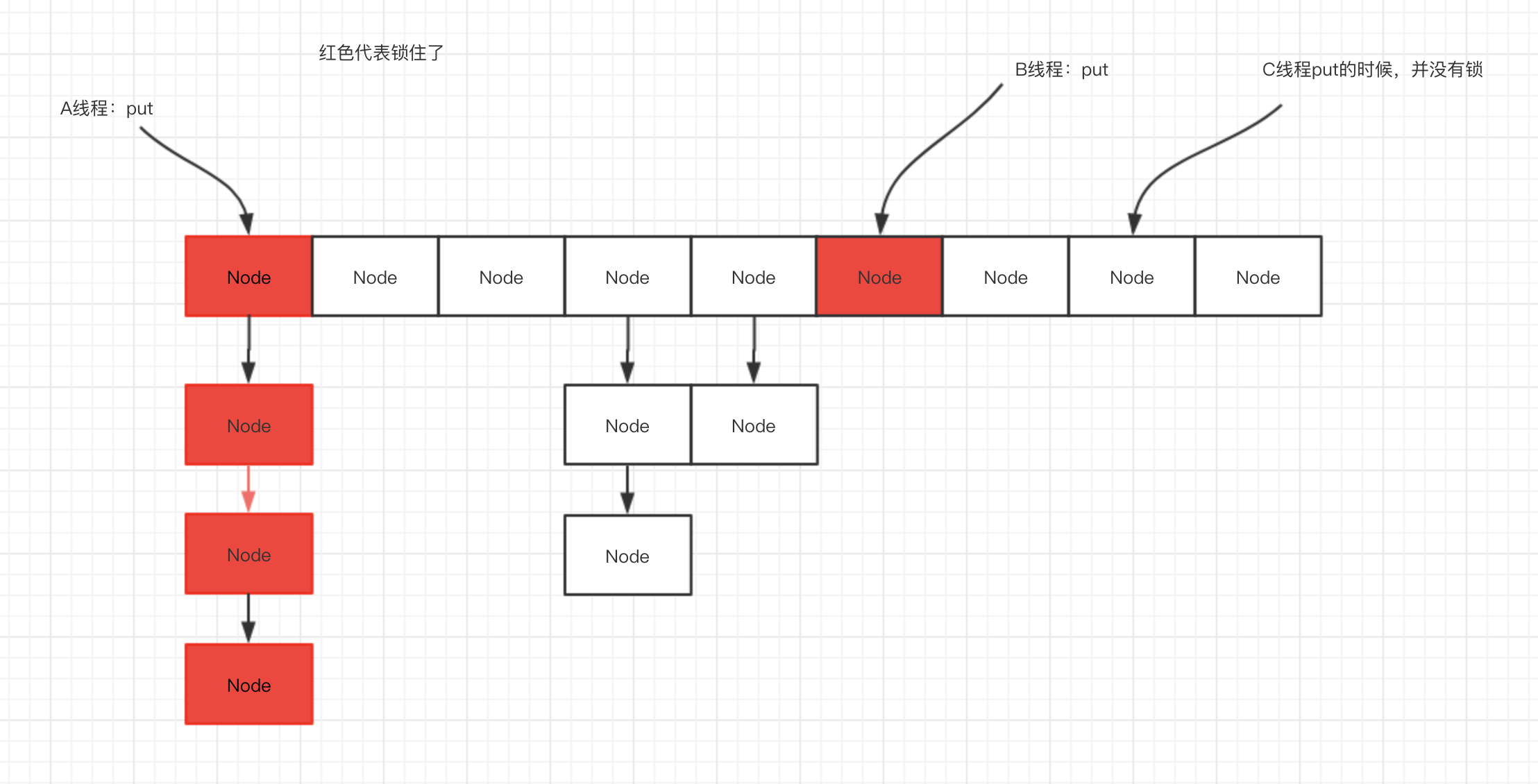1. 减小锁粒度：将Node链表的头节点作为锁，若在默认大小16情况下，将有16把锁，大大减小了锁竞争（上下文切换），就像开头所说，将串行的部分最大化缩小，在理想情况下线程的put操作都为并行操作。同时直接锁住头节点，保证了线程安全
2. Unsafe的getObjectVolatile方法：此方法确保获取到的值为最新

## 扩容操作的线程安全

``````private final void transfer(Node<K,V>[] tab, Node<K,V>[] nextTab) {

int n = tab.length, stride;
//根据机器CPU核心数来计算，一条线程负责Node数组中多长的迁移量
if ((stride = (NCPU > 1) ? (n >>> 3) / NCPU : n) < MIN_TRANSFER_STRIDE)
//本线程分到的迁移量
//假设为16（默认也为16）
stride = MIN_TRANSFER_STRIDE; // subdivide range
//nextTab若为空代表线程是第一个进行迁移的
//初始化迁移后的新Node数组
if (nextTab == null) {
// initiating
try {

@SuppressWarnings("unchecked")
//这里n为旧数组长度，左移一位相当于乘以2
//例如原数组长度16，新数组长度则为32
Node<K,V>[] nt = (Node<K,V>[])new Node<?,?>[n << 1];
nextTab = nt;
} catch (Throwable ex) {
// try to cope with OOME
sizeCtl = Integer.MAX_VALUE;
return;
}
//设置nextTable变量为新数组
nextTable = nextTab;
//假设为16
transferIndex = n;
}
//假设为32
int nextn = nextTab.length;
//标示Node对象，此对象的hash变量为-1
//在get或者put时若遇到此Node，则可以知道当前Node正在迁移
//传入nextTab对象
ForwardingNode<K,V> fwd = new ForwardingNode<K,V>(nextTab);
boolean finishing = false; // to ensure sweep before committing nextTab
for (int i = 0, bound = 0;;) {

Node<K,V> f; int fh;

int nextIndex, nextBound;
//i为当前正在处理的Node数组下标，每次处理一个Node节点就会自减1
if (--i >= bound || finishing)
//假设nextIndex=16
else if ((nextIndex = transferIndex) <= 0) {

i = -1;
}
//由以上假设，nextBound就为0
//且将nextIndex设置为0
else if (U.compareAndSwapInt
(this, TRANSFERINDEX, nextIndex,
nextBound = (nextIndex > stride ?
nextIndex - stride : 0))) {

//bound=0
bound = nextBound;
//i=16-1=15
i = nextIndex - 1;
}
}
if (i < 0 || i >= n || i + n >= nextn) {

int sc;
if (finishing) {

nextTable = null;
table = nextTab;
sizeCtl = (n << 1) - (n >>> 1);
return;
}
if (U.compareAndSwapInt(this, SIZECTL, sc = sizeCtl, sc - 1)) {

if ((sc - 2) != resizeStamp(n) << RESIZE_STAMP_SHIFT)
return;
i = n; // recheck before commit
}
}
//此时i=15，取出Node数组下标为15的那个Node，若为空则不需要迁移
//直接设置占位标示，代表此Node已处理完成
else if ((f = tabAt(tab, i)) == null)
advance = casTabAt(tab, i, null, fwd);
//检测此Node的hash是否为MOVED，MOVED是一个常量-1，也就是上面说的占位Node的hash
//如果是占位Node，证明此节点已经处理过了，跳过i=15的处理，继续循环
else if ((fh = f.hash) == MOVED)
else {

//锁住这个Node
synchronized (f) {

//确认Node是原先的Node
if (tabAt(tab, i) == f) {

//ln为lowNode，低位Node，hn为highNode，高位Node
//这两个概念下面以图来说明
Node<K,V> ln, hn;
if (fh >= 0) {

//此时fh与原来Node数组长度进行与运算
//如果高X位为0，此时runBit=0
//如果高X位为1，此时runBit=1
int runBit = fh & n;
Node<K,V> lastRun = f;
for (Node<K,V> p = f.next; p != null; p = p.next) {

//这里的Node，都是同一Node链表中的Node对象
int b = p.hash & n;
if (b != runBit) {

runBit = b;
lastRun = p;
}
}
//正如上面所说，runBit=0，表示此Node为低位Node
if (runBit == 0) {

ln = lastRun;
hn = null;
}
else {

//Node为高位Node
hn = lastRun;
ln = null;
}
for (Node<K,V> p = f; p != lastRun; p = p.next) {

int ph = p.hash; K pk = p.key; V pv = p.val;
//若hash和n与运算为0，证明为低位Node，原理同上
if ((ph & n) == 0)
ln = new Node<K,V>(ph, pk, pv, ln);
//这里将高位Node与地位Node都各自组成了两个链表
else
hn = new Node<K,V>(ph, pk, pv, hn);
}
//将低位Node设置到新Node数组中，下标为原来的位置
setTabAt(nextTab, i, ln);
//将高位Node设置到新Node数组中，下标为原来的位置加上原Node数组长度
setTabAt(nextTab, i + n, hn);
//将此Node设置为占位Node，代表处理完成
setTabAt(tab, i, fwd);
//继续循环
}
....
}
}
}
}
}
``````

``````//根据key的hashCode再散列
//使用(n - 1) & hash 运算，定位Node数组中下标值
(f = tabAt(tab, i = (n - 1) & hash);
``````

• （16 - 1）和 9 进行与运算 -> 0000 1111 和 0000 1001 结果还是 0000 1001 = 9

• （32 - 1）和 9 进行与运算 -> 0001 1111 和 0000 1001 结果还是9

• （16 - 1）和 20 进行与运算 -> 0000 1111 和 0001 0100 结果是 0000 0100 = 4
• （32 - 1）和 20 进行与运算 -> 0001 1111 和 0001 0100 结果是 0001 0100 = 20

``````for (Node<K,V> p = f; p != lastRun; p = p.next) {

int ph = p.hash;
K pk = p.key;
V pv = p.val;
if ((ph & n) == 0)
ln = new Node<K,V>(ph, pk, pv, ln);
else
hn = new Node<K,V>(ph, pk, pv, hn);
}
``````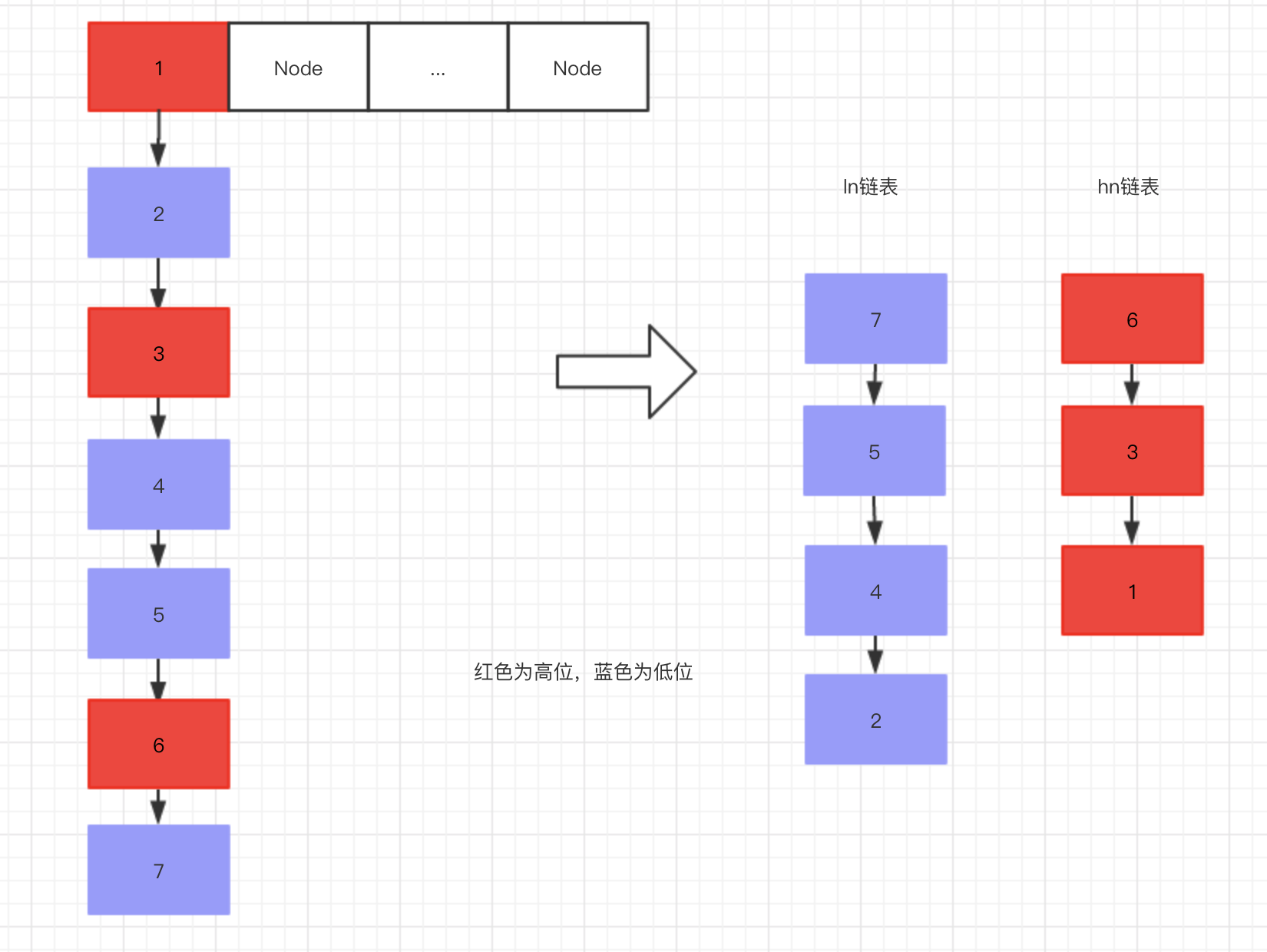``````setTabAt(nextTab, i, ln);
setTabAt(nextTab, i + n, hn);
``````

### 扩容时的get操作

``````public V get(Object key) {

Node<K,V>[] tab; Node<K,V> e, p; int n, eh; K ek;
if ((tab = table) != null && (n = tab.length) > 0 &&
(e = tabAt(tab, (n - 1) & h)) != null) {

if ((eh = e.hash) == h) {

if ((ek = e.key) == key || (ek != null && key.equals(ek)))
return e.val;
}
//假如Node节点的hash值小于0
//则有可能是fwd节点
else if (eh < 0)
//调用节点对象的find方法查找值
return (p = e.find(h, key)) != null ? p.val : null;
while ((e = e.next) != null) {

if (e.hash == h &&
((ek = e.key) == key || (ek != null && key.equals(ek))))
return e.val;
}
}
return null;
}
``````

``````//内部类 ForwardingNode中
Node<K,V> find(int h, Object k) {

// loop to avoid arbitrarily deep recursion on forwarding nodes
// 这里的查找，是去新Node数组中查找的
// 下面的查找过程与HashMap查找无异，不多赘述
outer: for (Node<K,V>[] tab = nextTable;;) {

Node<K,V> e; int n;
if (k == null || tab == null || (n = tab.length) == 0 ||
(e = tabAt(tab, (n - 1) & h)) == null)
return null;
for (;;) {

int eh; K ek;
if ((eh = e.hash) == h &&
((ek = e.key) == k || (ek != null && k.equals(ek))))
return e;
if (eh < 0) {

if (e instanceof ForwardingNode) {

tab = ((ForwardingNode<K,V>)e).nextTable;
continue outer;
}
else
return e.find(h, k);
}
if ((e = e.next) == null)
return null;
}
}
}
``````

### 多线程协助扩容

``````final V putVal(K key, V value, boolean onlyIfAbsent) {

if (key == null || value == null) throw new NullPointerException();
int binCount = 0;
for (Node<K,V>[] tab = table;;) {

Node<K,V> f; int n, i, fh;
if (tab == null || (n = tab.length) == 0)
tab = initTable();
else if ((f = tabAt(tab, i = (n - 1) & hash)) == null) {

if (casTabAt(tab, i, null,
new Node<K,V>(hash, key, value, null)))
break;                   // no lock when adding to empty bin
}
//若此时发现了占位Node，证明此时HashMap正在迁移
else if ((fh = f.hash) == MOVED)
//进行协助迁移
tab = helpTransfer(tab, f);
...
}
``````
``````final Node<K,V>[] helpTransfer(Node<K,V>[] tab, Node<K,V> f) {

Node<K,V>[] nextTab; int sc;
if (tab != null && (f instanceof ForwardingNode) &&
(nextTab = ((ForwardingNode<K,V>)f).nextTable) != null) {

int rs = resizeStamp(tab.length);
while (nextTab == nextTable && table == tab &&
(sc = sizeCtl) < 0) {

if ((sc >>> RESIZE_STAMP_SHIFT) != rs || sc == rs + 1 ||
sc == rs + MAX_RESIZERS || transferIndex <= 0)
break;
//sizeCtl加一，标示多一个线程进来协助扩容
if (U.compareAndSwapInt(this, SIZECTL, sc, sc + 1)) {

//扩容
transfer(tab, nextTab);
break;
}
}
return nextTab;
}
return table;
}
``````

``````1000 0000 0001 1011 0000 0000 0000 0010
``````

### 在什么情况下会进行扩容操作？

• 在put值时，发现Node为占位Node（fwd）时，会协助扩容

• 在新增节点后，检测到链表长度大于8时

``````final V putVal(K key, V value, boolean onlyIfAbsent) {

...
if (binCount != 0) {

//TREEIFY_THRESHOLD=8，当链表长度大于8时
if (binCount >= TREEIFY_THRESHOLD)
//调用treeifyBin方法
treeifyBin(tab, i);
if (oldVal != null)
return oldVal;
break;
}
...
}
``````

treeifyBin方法会将链表转换为红黑树，增加查找效率，但在这之前，会检查数组长度，若小于64，则会优先做扩容操作：

``````private final void treeifyBin(Node<K,V>[] tab, int index) {

Node<K,V> b; int n, sc;
if (tab != null) {

//MIN_TREEIFY_CAPACITY=64
//若数组长度小于64，则先扩容
if ((n = tab.length) < MIN_TREEIFY_CAPACITY)
//扩容
tryPresize(n << 1);
else if ((b = tabAt(tab, index)) != null && b.hash >= 0) {

synchronized (b) {

//...转换为红黑树的操作
}
}
}
}
``````

``````final V putVal(K key, V value, boolean onlyIfAbsent) {

...
//在下面一节会讲到，此方法统计容器元素数量
return null;
}
``````
``````private final void addCount(long x, int check) {

CounterCell[] as; long b, s;
if ((as = counterCells) != null ||
!U.compareAndSwapLong(this, BASECOUNT, b = baseCount, s = b + x)) {

//统计元素个数的操作...
}
if (check >= 0) {

Node<K,V>[] tab, nt; int n, sc;
//元素个数达到阈值，进行扩容
while (s >= (long)(sc = sizeCtl) && (tab = table) != null &&
(n = tab.length) < MAXIMUM_CAPACITY) {

int rs = resizeStamp(n);
//发现sizeCtl为负数，证明有线程正在迁移
if (sc < 0) {

if ((sc >>> RESIZE_STAMP_SHIFT) != rs || sc == rs + 1 ||
sc == rs + MAX_RESIZERS || (nt = nextTable) == null ||
transferIndex <= 0)
break;
if (U.compareAndSwapInt(this, SIZECTL, sc, sc + 1))
transfer(tab, nt);
}
//不为负数，则为第一个迁移的线程
else if (U.compareAndSwapInt(this, SIZECTL, sc,
(rs << RESIZE_STAMP_SHIFT) + 2))
transfer(tab, null);
s = sumCount();
}
}
}
``````

### 总结

ConcurrentHashMap运用各类CAS操作，将扩容操作的并发性能实现最大化，在扩容过程中，就算有线程调用get查询方法，也可以安全的查询数据，若有线程进行put操作，还会协助扩容，利用sizeCtl标记位和各种volatile变量进行CAS操作达到多线程之间的通信、协助，在迁移过程中只锁一个Node节点，即保证了线程安全，又提高了并发性能。

## 统计容器大小的线程安全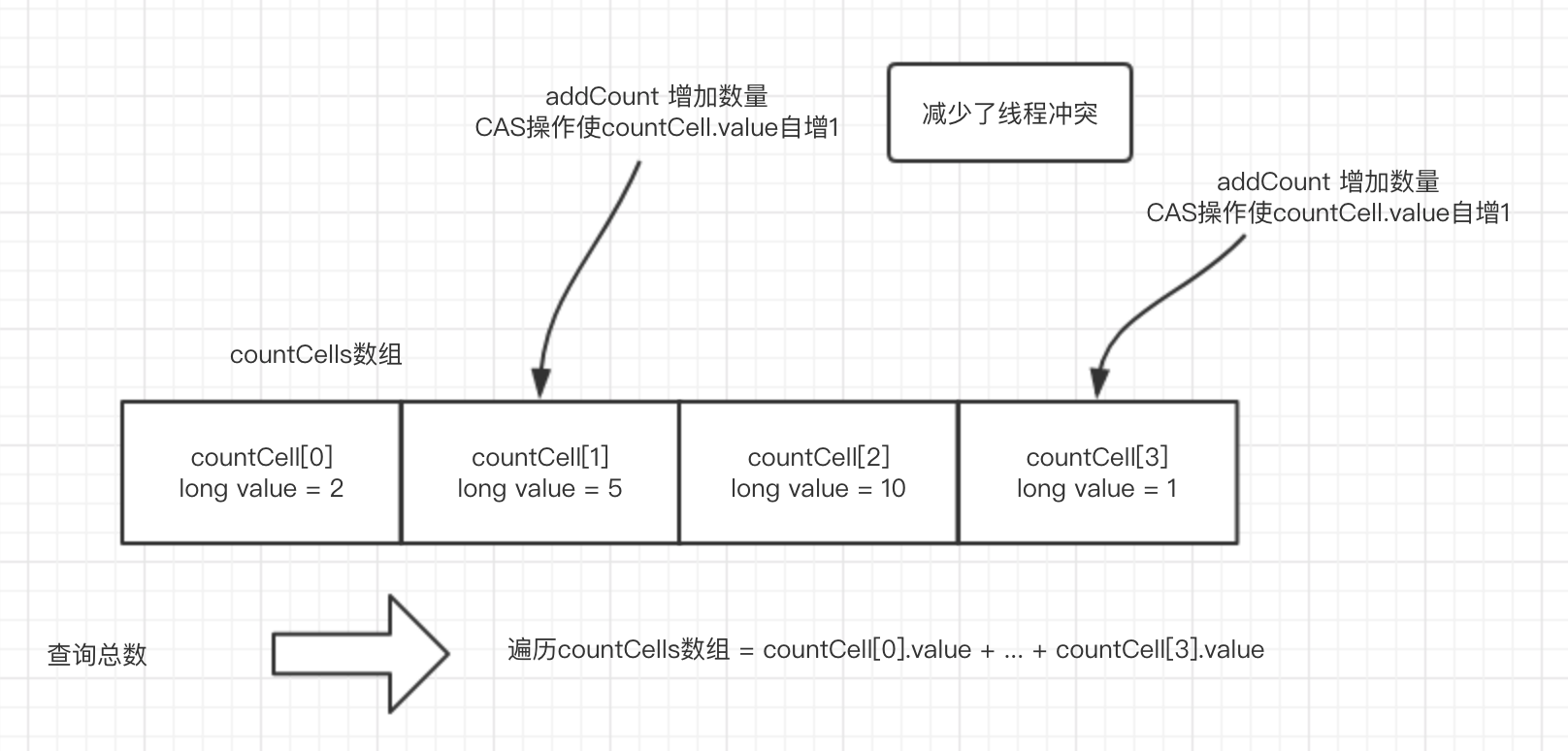``````@sun.misc.Contended static final class CounterCell {

volatile long value;
CounterCell(long x) {
value = x; }
}
``````

``````//计数，并检查长度是否达到阈值
private final void addCount(long x, int check) {

//计数桶
CounterCell[] as; long b, s;
//如果counterCells不为null，则代表已经初始化了，直接进入if语句块
//若竞争不严重，counterCells有可能还未初始化，为null，先尝试CAS操作递增baseCount值
if ((as = counterCells) != null ||
!U.compareAndSwapLong(this, BASECOUNT, b = baseCount, s = b + x)) {

//进入此语句块有两种可能
//1.counterCells被初始化完成了，不为null
//2.CAS操作递增baseCount值失败了，说明有竞争
CounterCell a; long v; int m;
//标志是否存在竞争
boolean uncontended = true;
//1.先判断计数桶是否还没初始化，则as=null，进入语句块
//2.判断计数桶长度是否为空或，若是进入语句块
//3.这里做了一个线程变量随机数，与上桶大小-1，若桶的这个位置为空，进入语句块
//4.到这里说明桶已经初始化了，且随机的这个位置不为空，尝试CAS操作使桶加1，失败进入语句块
if (as == null || (m = as.length - 1) < 0 ||
(a = as[ThreadLocalRandom.getProbe() & m]) == null ||
!(uncontended =
U.compareAndSwapLong(a, CELLVALUE, v = a.value, v + x))) {

return;
}
if (check <= 1)
return;
//统计容器大小
s = sumCount();
}
...
}
``````

### 假设当前线程为第一个put的线程

``````if ((as = counterCells) != null ||
!U.compareAndSwapLong(this, BASECOUNT, b = baseCount, s = b + x))
``````

``````private transient volatile long baseCount;
``````

``````final long sumCount() {

//获取计数桶
CounterCell[] as = counterCells; CounterCell a;
//获取baseCount，赋值给sum总数
long sum = baseCount;
//若计数桶不为空，统计计数桶内的值
if (as != null) {

for (int i = 0; i < as.length; ++i) {

//遍历计数桶，将value值相加
if ((a = as[i]) != null)
sum += a.value;
}
}
return sum;
}
``````

1. CAS方式直接递增：在线程竞争不大的时候，直接使用CAS操作递增baseCount值即可，这里说的竞争不大指的是CAS操作不会失败的情况
2. 分而治之桶计数：若出现了CAS操作失败的情况，则证明此时有线程竞争了，计数方式从CAS方式转变为分而治之的桶计数方式

### 出现了线程竞争导致CAS失败

``````   private final void fullAddCount(long x, boolean wasUncontended) {

int h;
if ((h = ThreadLocalRandom.getProbe()) == 0) {

wasUncontended = true;
}
boolean collide = false;                // True if last slot nonempty
for (;;) {

CounterCell[] as; CounterCell a; int n; long v;
...
//如果计数桶!=null，证明已经初始化，此时不走此语句块
if ((as = counterCells) != null && (n = as.length) > 0) {

...
}
//进入此语句块进行计数桶的初始化
//CAS设置cellsBusy=1，表示现在计数桶Busy中...
else if (cellsBusy == 0 && counterCells == as &&
U.compareAndSwapInt(this, CELLSBUSY, 0, 1)) {

//若有线程同时初始化计数桶，由于CAS操作只有一个线程进入这里
boolean init = false;
try {
// Initialize table
//再次确认计数桶为空
if (counterCells == as) {

//初始化一个长度为2的计数桶
CounterCell[] rs = new CounterCell;
//h为一个随机数，与上1则代表结果为0、1中随机的一个
//也就是在0、1下标中随便选一个计数桶，x=1，放入1的值代表增加1个容量
rs[h & 1] = new CounterCell(x);
//将初始化好的计数桶赋值给ConcurrentHashMap
counterCells = rs;
init = true;
}
} finally {

//最后将busy标识设置为0，表示不busy了
cellsBusy = 0;
}
if (init)
break;
}
//若有线程同时来初始化计数桶，则没有抢到busy资格的线程就先来CAS递增baseCount
else if (U.compareAndSwapLong(this, BASECOUNT, v = baseCount, v + x))
break;                          // Fall back on using base
}
}
``````

### 计数桶扩容

1. 什么条件下会进行计数桶的扩容？
2. 扩容操作是怎么样的？

``````private final void addCount(long x, int check) {

CounterCell[] as; long b, s;
if ((as = counterCells) != null ||
!U.compareAndSwapLong(this, BASECOUNT, b = baseCount, s = b + x)) {

CounterCell a; long v; int m;
boolean uncontended = true;
//此时显然会在计数桶数组中随机选一个计数桶
//然后使用CAS操作将此计数桶中的value+1
if (as == null || (m = as.length - 1) < 0 ||
(a = as[ThreadLocalRandom.getProbe() & m]) == null ||
!(uncontended =
U.compareAndSwapLong(a, CELLVALUE, v = a.value, v + x))) {

return;
}
if (check <= 1)
return;
s = sumCount();
}
...
}
``````

``````private final void fullAddCount(long x, boolean wasUncontended) {

int h;
if ((h = ThreadLocalRandom.getProbe()) == 0) {

wasUncontended = true;
}
boolean collide = false;                // True if last slot nonempty
for (;;) {

CounterCell[] as; CounterCell a; int n; long v;
//计数桶初始化好了，一定是走这个if语句块
if ((as = counterCells) != null && (n = as.length) > 0) {

//从计数桶数组随机选一个计数桶，若为null表示该桶位还没线程递增过
if ((a = as[(n - 1) & h]) == null) {

//查看计数桶busy状态是否被标识
if (cellsBusy == 0) {
// Try to attach new Cell
//若不busy，直接new一个计数桶
CounterCell r = new CounterCell(x); // Optimistic create
//CAS操作，标示计数桶busy中
if (cellsBusy == 0 &&
U.compareAndSwapInt(this, CELLSBUSY, 0, 1)) {

boolean created = false;
try {
// Recheck under lock
CounterCell[] rs; int m, j;
//在锁下再检查一次计数桶为null
if ((rs = counterCells) != null &&
(m = rs.length) > 0 &&
rs[j = (m - 1) & h] == null) {

//将刚刚创建的计数桶赋值给对应位置
rs[j] = r;
created = true;
}
} finally {

//标示不busy了
cellsBusy = 0;
}
if (created)
break;
continue;           // Slot is now non-empty
}
}
collide = false;
}
else if (!wasUncontended)       // CAS already known to fail
wasUncontended = true;      // Continue after rehash
//走到这里代表计数桶不为null，尝试递增计数桶
else if (U.compareAndSwapLong(a, CELLVALUE, v = a.value, v + x))
break;
else if (counterCells != as || n >= NCPU)
collide = false;            // At max size or stale
//若CAS操作失败了，到了这里，会先进入一次，然后再走一次刚刚的for循环
//若是第二次for循环，collide=true，则不会走进去
else if (!collide)
collide = true;
//计数桶扩容，一个线程若走了两次for循环，也就是进行了多次CAS操作递增计数桶失败了
//则进行计数桶扩容，CAS标示计数桶busy中
else if (cellsBusy == 0 &&
U.compareAndSwapInt(this, CELLSBUSY, 0, 1)) {

try {

//确认计数桶还是同一个
if (counterCells == as) {
// Expand table unless stale
//将长度扩大到2倍
CounterCell[] rs = new CounterCell[n << 1];
//遍历旧计数桶，将引用直接搬过来
for (int i = 0; i < n; ++i)
rs[i] = as[i];
//赋值
counterCells = rs;
}
} finally {

//取消busy状态
cellsBusy = 0;
}
collide = false;
continue;                   // Retry with expanded table
}
}
else if (cellsBusy == 0 && counterCells == as &&
U.compareAndSwapInt(this, CELLSBUSY, 0, 1)) {

//初始化计数桶...
}
else if (U.compareAndSwapLong(this, BASECOUNT, v = baseCount, v + x))
break;                          // Fall back on using base
}
}
``````

1. 什么条件下会进行计数桶的扩容？

答：在CAS操作递增计数桶失败了3次之后，会进行扩容计数桶操作，注意此时同时进行了两次随机定位计数桶来进行CAS递增的，所以此时可以保证大概率是因为计数桶不够用了，才会进行计数桶扩容

2. 扩容操作是怎么样的？

答：计数桶长度增加到两倍长度，数据直接遍历迁移过来，由于计数桶不像HashMap数据结构那么复杂，有hash算法的影响，加上计数桶只是存放一个long类型的计数值而已，所以直接赋值引用即可。

### 总结

1. 利用CAS递增baseCount值来感知是否存在线程竞争，若竞争不大直接CAS递增baseCount值即可，性能与直接baseCount++差别不大
2. 若存在线程竞争，则初始化计数桶，若此时初始化计数桶的过程中也存在竞争，多个线程同时初始化计数桶，则没有抢到初始化资格的线程直接尝试CAS递增baseCount值的方式完成计数，最大化利用了线程的并行。此时使用计数桶计数，分而治之的方式来计数，此时两个计数桶最大可提供两个线程同时计数，同时使用CAS操作来感知线程竞争，若两个桶情况下CAS操作还是频繁失败（失败3次），则直接扩容计数桶，变为4个计数桶，支持最大同时4个线程并发计数，以此类推…同时使用位运算和随机数的方式"负载均衡"一样的将线程计数请求接近均匀的落在各个计数桶中。

## get操作的线程安全

``````public V get(Object key) {

Node<K,V>[] tab; Node<K,V> e, p; int n, eh; K ek;
//此过程与HashMap的get操作无异，不多赘述
if ((tab = table) != null && (n = tab.length) > 0 &&
(e = tabAt(tab, (n - 1) & h)) != null) {

if ((eh = e.hash) == h) {

if ((ek = e.key) == key || (ek != null && key.equals(ek)))
return e.val;
}
//当hash<0,有可能是在迁移,使用fwd占位Node去查找新table中的数据
else if (eh < 0)
return (p = e.find(h, key)) != null ? p.val : null;
while ((e = e.next) != null) {

if (e.hash == h &&
((ek = e.key) == key || (ek != null && key.equals(ek))))
return e.val;
}
}
return null;
}
``````

``````static final <K,V> Node<K,V> tabAt(Node<K,V>[] tab, int i) {

return (Node<K,V>)U.getObjectVolatile(tab, ((long)i << ASHIFT) + ABASE);
}
``````

## JDK1.7与1.8的不同实现

JDK1.7的ConcurrentHashMap底层数据结构：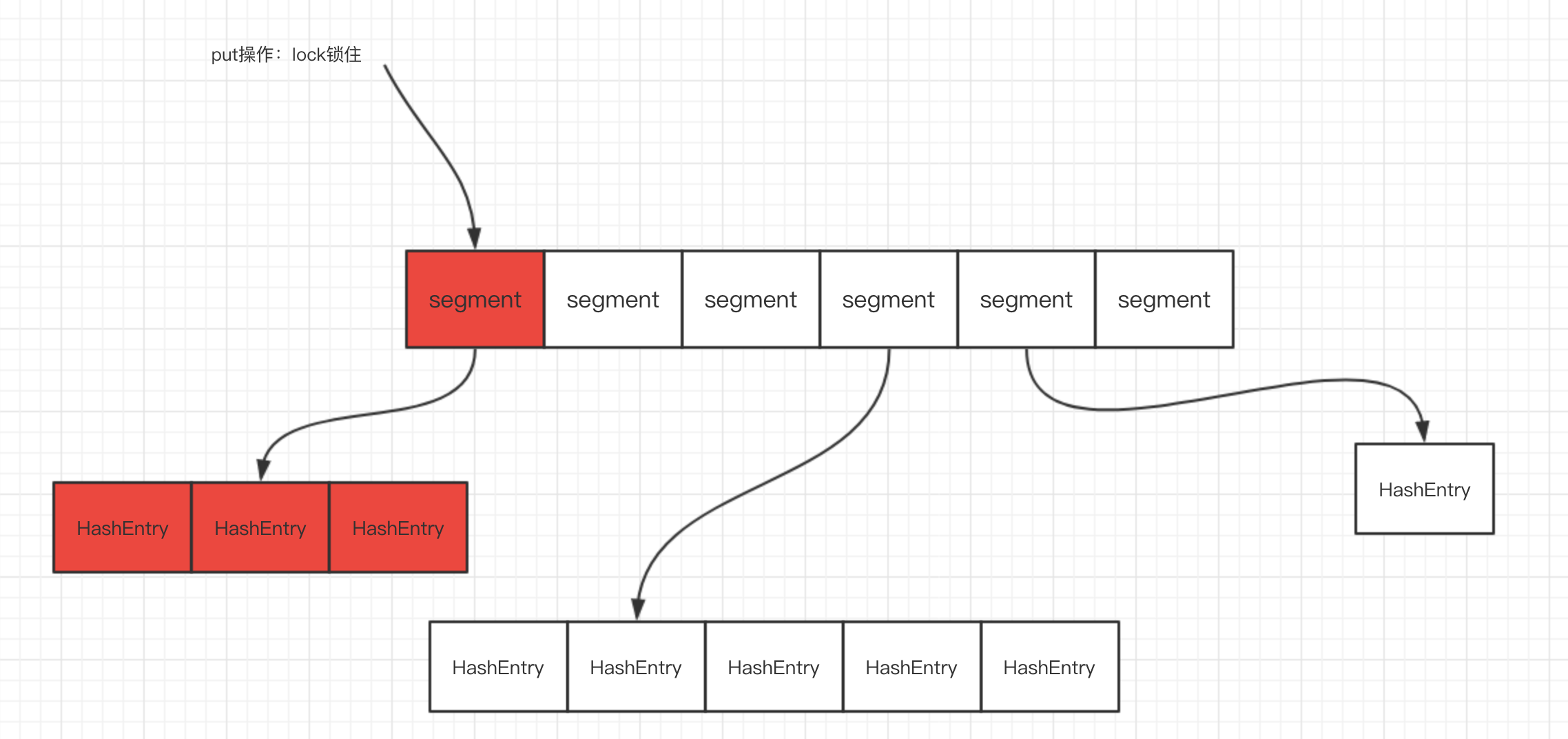## 总结

————————————————

wski

• 浏览更多精彩评论
• 和开发者讨论交流，共同进步

## 为你推荐## 美团面试官：可重复读隔离级别实现原理是什么？（一文搞懂MVCC机制）## 我坚定的认为，这个源码肯定是有 BUG 的！## 各个语言运行100万个并发任务需要多少内存？## 从CPU的视角看 多线程代码为什么那么难写！